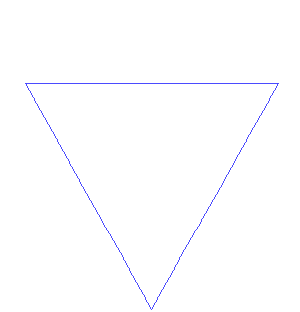# Fun Science: Fractals in Nature and Fractal Measurement

This post continues Wednesday’s post about fractals and the Mandelbrot set. Fractals are a branch of mathematics that we can observe in our daily life. Something is said to be fractal when a small piece of an object resembles a larger part of itself. The featured image is of romanesco broccoli; as you can see, each small cone on the broccoli resembles the overall structure of the vegetable. For this reason, the mathematical terms “fractal” and “self-similar” are closely related.

Examples of fractals in nature abound. The heartbeat of a healthy person is fractal when plotted in time; interestingly, people with various health problems show less fractal character to their heart rate. For a great slide show with images of fractal-ness in nature, check out this Wired article. Fractals have been observed in ocean waves, mountain structures, fern, lightning, city layout, seashell, trees, and many others. Many computer graphics of natural phenomena are generated using fractal processes.Koch Snowflake (Wikipedia)

The Koch snowflake (above), is a fractal generated from a line. As the fractal pattern is repeated, the length of the curve grows infinite. A line segment does not have infinite length, and yet the Koch Snowflake clearly does not fill space. So what is the dimension of this object? Through a method called the “box counting method“, we can determine the dimensionality of a fractal object. The box counting method is used to estimate area and coastal length from satellite pictures, as demonstrated below.

In short, we can see how the number of boxes needed to define a length or space changes as the box size changes. For a line, the number of boxes needed grows as 1n. For a space, the number of boxes grows as 2n. The method is explained in more detail here. Intuitively, we can tell the Koch Snowflake has a dimension between 1 and 2. It turns out that, using the Box Counting method, we can determine that the Koch Snowflake has a fractal dimension of log(4)/log(3), or about 1.26.

Fractal dimensions turn up in strange places. For example, chaotic attractors have fractal dimension. The Lorenz attractor, above, has a fractal dimension of 2.06. In the future I will discuss chaos and chaotic attractors. Check out my previous science posts on synchrony and art resembling science.